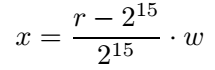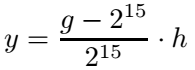SMV: Sequences with Motion Vectors

Each sequence contains the following information:

• Raw sequence in YUV420 format,
• Motion and depth information for each frame in TIFF-format (with respect to the previous frame),
• A cfg-file containing sequence information in the HM-configuration file format.

In the TIFF-files the information is stored in the following format:

• R-channel: x-motion
• G-channel: y-mtion
• B-channel: z-motion (in depth domain, normalized to the range [0, 1])
• Alpha-channel: depth (in the same domain as the z-motion)

Each channel is saved in 16 bits bit depth. If r and g are the color values of one image pixel (in the range [0, 2^16]), the corresponding motion in pixel domain (x,y) is calculated byandwhere w and h are the frame width and height, respectively. The normalization to the frame dimensions ensures that the maximum MV length can always be obtained. For all sequences, the motion information keeps quarter-pel accuracy.

In C++, the tiff-files can be read using the freely available libtiff library.